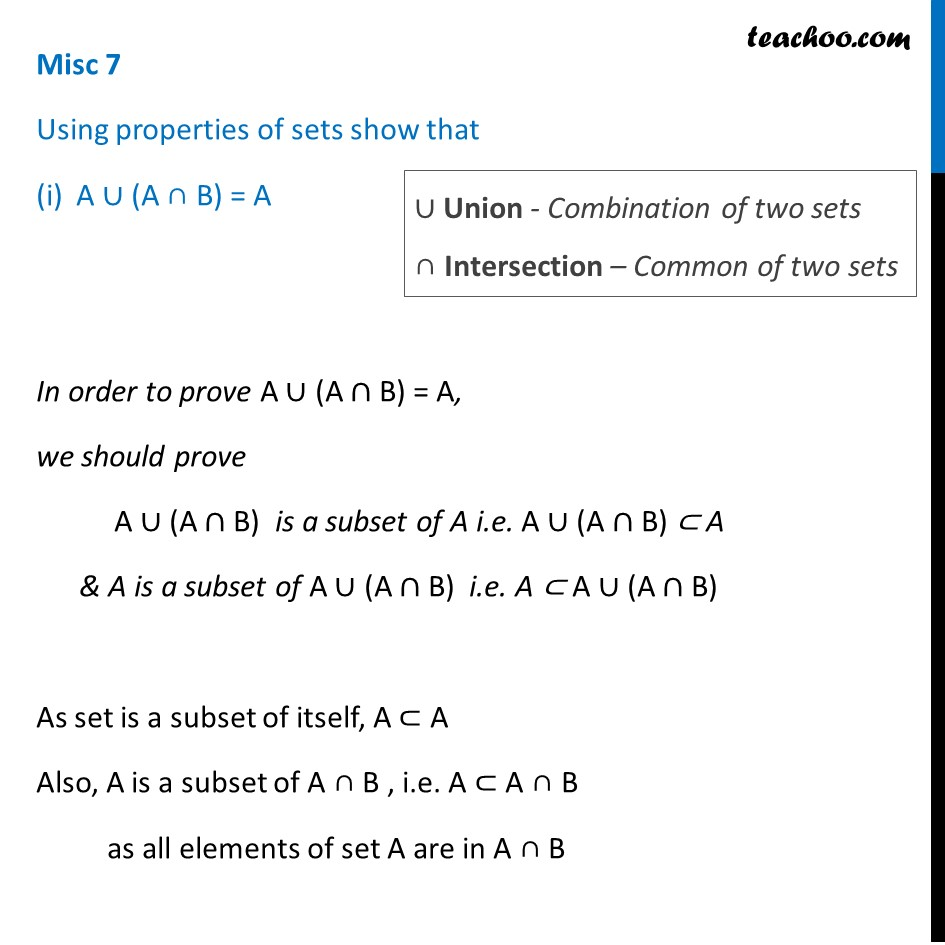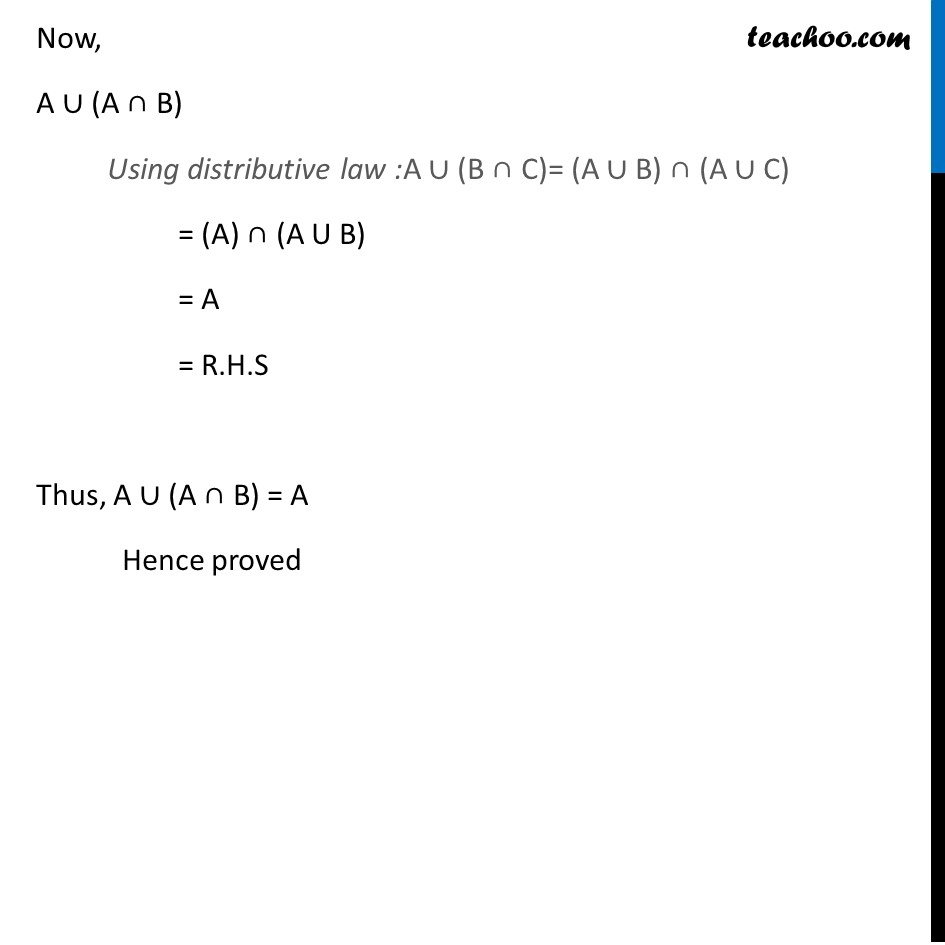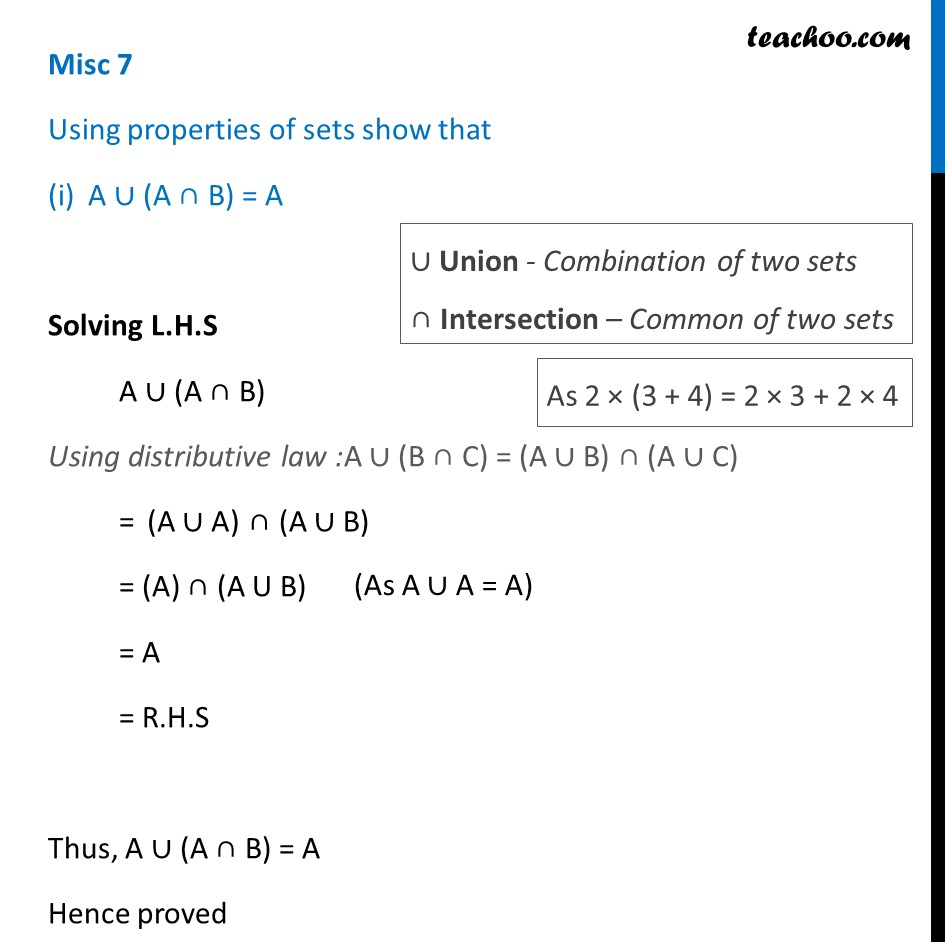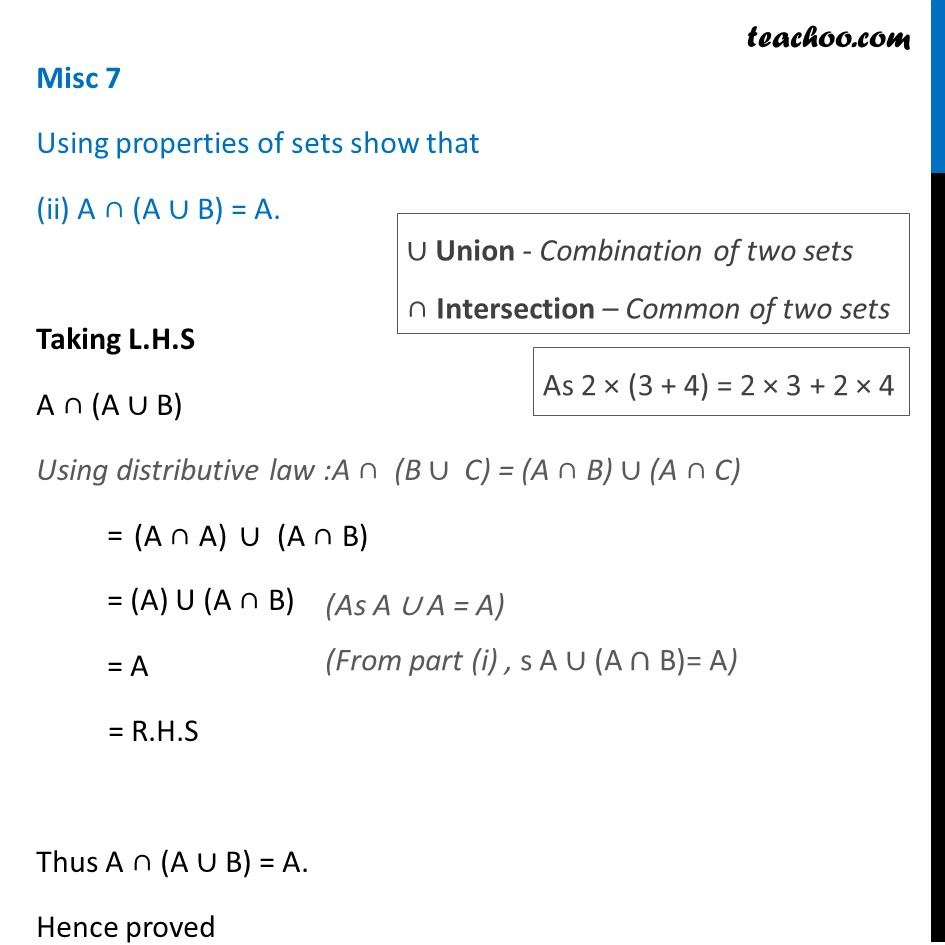Miscellaneous

Chapter 1 Class 11 Sets
Serial order wiseLearn in your speed, with individual attention - Teachoo Maths 1-on-1 Class

### Transcript

Misc 7 Using properties of sets show that A ∪ (A ∩ B) = A In order to prove A ∪ (A ∩ B) = A, we should prove A ∪ (A ∩ B) is a subset of A i.e. A ∪ (A ∩ B) ⊂ A & A is a subset of A ∪ (A ∩ B) i.e. A ⊂ A ∪ (A ∩ B) As set is a subset of itself, A ⊂ A Also, A is a subset of A ∩ B , i.e. A ⊂ A ∩ B as all elements of set A are in A ∩ B Now, A ∪ (A ∩ B) Using distributive law :A ∪ (B ∩ C)= (A ∪ B) ∩ (A ∪ C) = (A) ∩ (A U B) = A = R.H.S Thus, A ∪ (A ∩ B) = A Hence proved Misc 7 Using properties of sets show that A ∪ (A ∩ B) = A Solving L.H.S A ∪ (A ∩ B) Using distributive law :A ∪ (B ∩ C) = (A ∪ B) ∩ (A ∪ C) = = (A) ∩ (A U B) = A = R.H.S Thus, A ∪ (A ∩ B) = A Hence proved Misc 7 Using properties of sets show that (ii) A ∩ (A ∪ B) = A. Taking L.H.S A ∩ (A ∪ B) Using distributive law :A ∩ (B ∪ C) = (A ∩ B) ∪ (A ∩ C) = = (A) U (A ∩ B) = A = R.H.S Thus A ∩ (A ∪ B) = A. Hence proved